# Setup Guide

This tutorial is on how to set up the development environment for programming with the NM Dev library (the Java version). In order to use the NM Dev library, you need to know some Java programming. Sun (or Oracle) provides excellent online tutorials on learning Java. You can find a lot of easy-to-read-and-follow lessons in Sun’s Java Tutorials.

## IDE

You will need an Integrated Development Environment (IDE) to program NM Dev. Although you may choose any IDE you like, we recommend Netbeans. You can download it from here.To learn about how to use NetBeans, you will find a quick start tutorial here. The most important skill for you to learn is debugging. This is especially true for those who are converting from Matlab and R and are used to printing out values. NetBeans debugger allows you to easily inspect values, place conditional breakpoints, pause and resume your program and much more. A quick Google search gives these articles.

We cannot emphasize enough that it is imperative that you must master using a debugger to make your programming experience productive and enjoyable.

Hotkey is another tool that will increase your programming productivity. My favorite hotkey is Ctrl+Shift+F (or Alt+Shift+f if you are using windows). It makes my code look pretty and neat. A list of hotkeys is found here. You can customize them to your own taste by going to Netbeans -> Preferences -> Keymap (or Tools -> Options -> Keymap for Windows/Linux).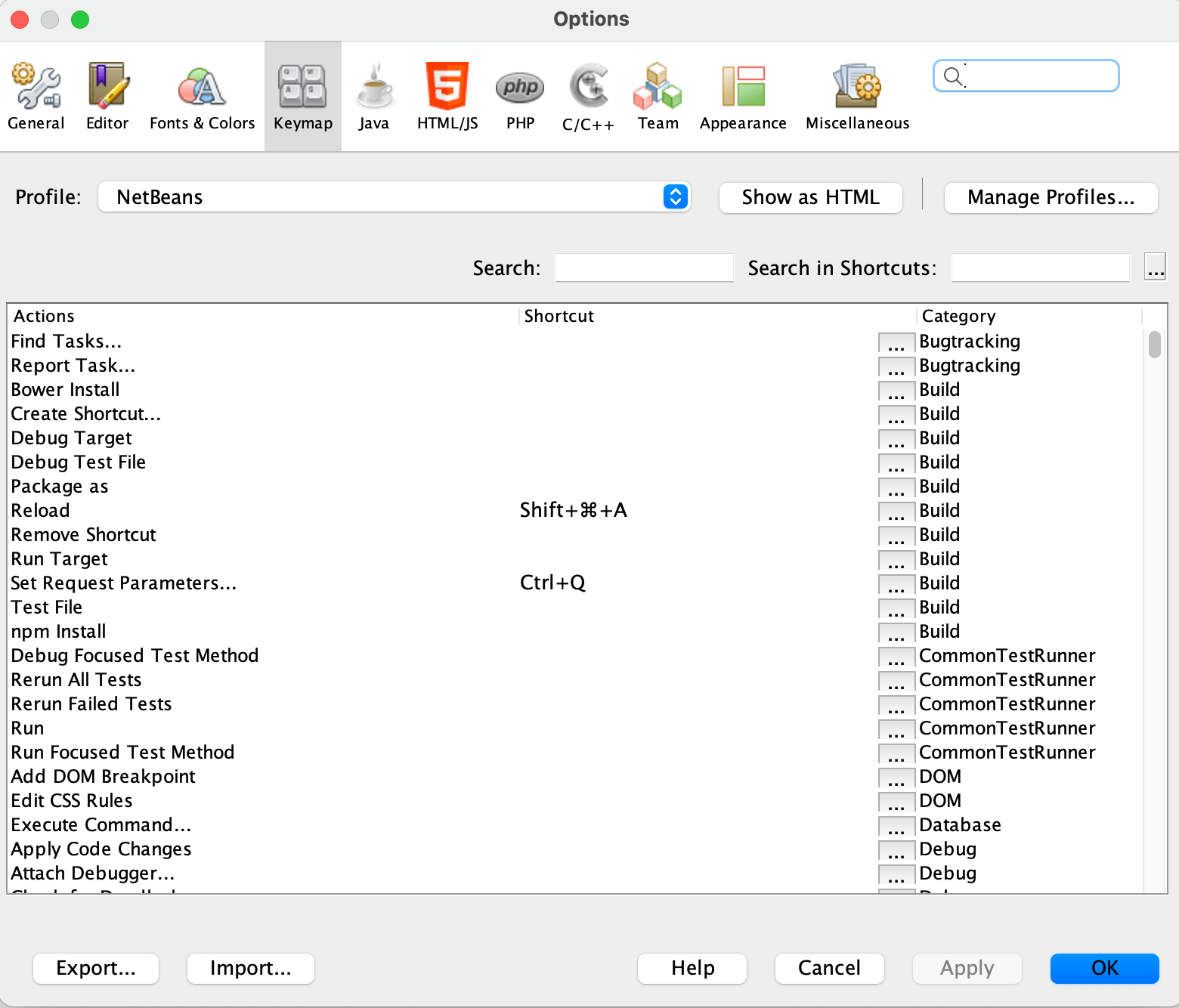## nmdev.jar

To program the NM Dev library, you will need a licensed copy of nmdev.jar (the actual file name depends on the version that you have). It is a compressed file that contains the NM Dev Java classes for numerical computing. To get a copy of the trial license, please email sales@nm.dev.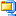nmdev-1.0.0.zip (13.1 MiB, 3,621 hits)

This section walks you through how to set up a NM Dev project. You may skip this section if you want to start programming NM Dev right away using the S2 IDE which comes in-built with NM Dev. Please read our Basic Trails to get started. If in the future you would like to update the NM Dev library for a newer version, you will need to come back to this section.

## Create a NM Dev project

To create a NM Dev project in NetBeans, open the NetBeans IDE, click File -> New Project… (Ctrl+Shift+N). In ‘categories’, choose ‘Java with Ant’; in projects, choose ‘Java Application’. Click ‘Next’.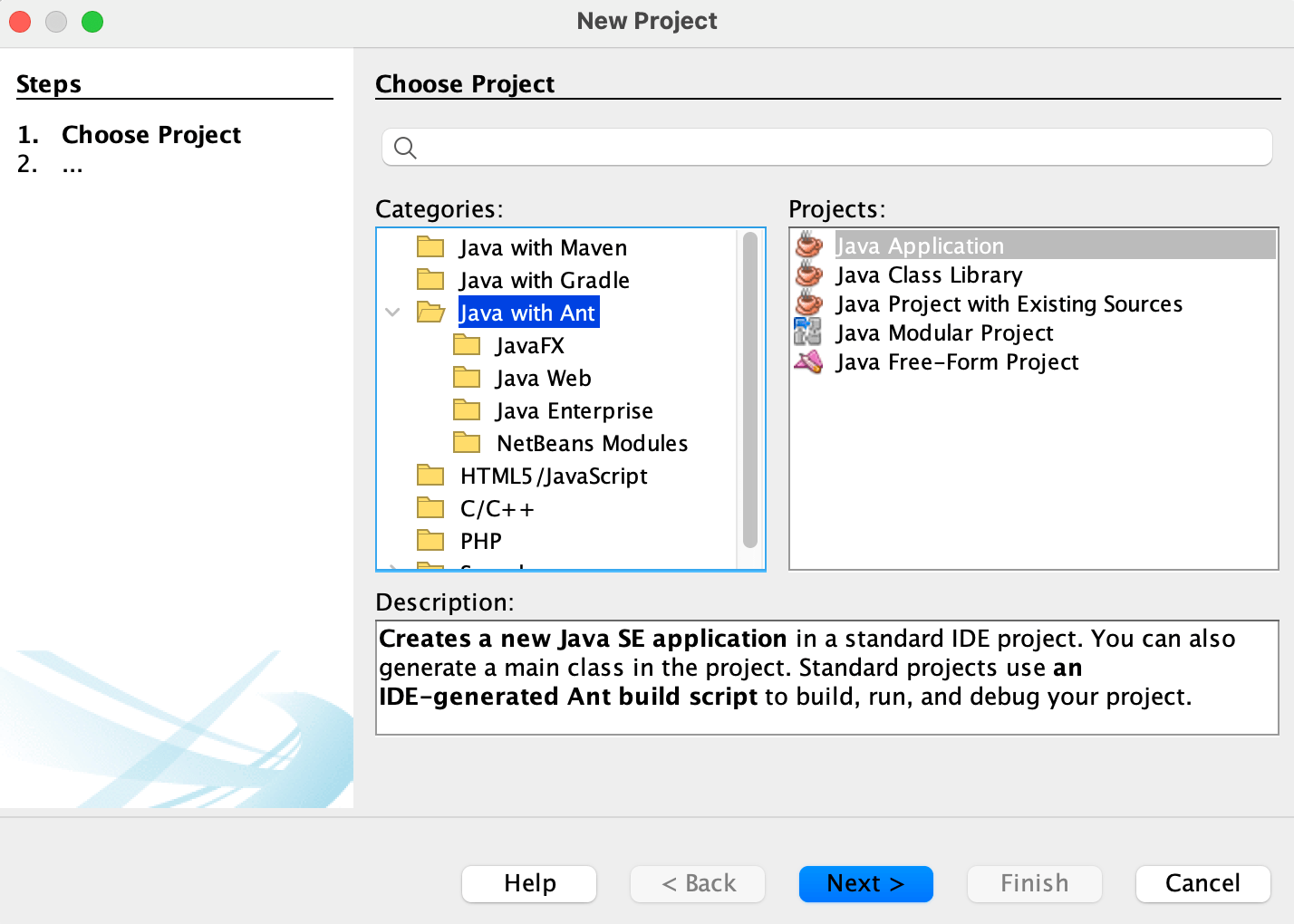In ‘Project Name’, type whatever you like, e.g., HelloNMDev. In ‘Project Location’, type where you would like to save your project. Make sure the box ‘Use dedicated Folder for Storing Libraries’ is checked.Then click ‘Finish’.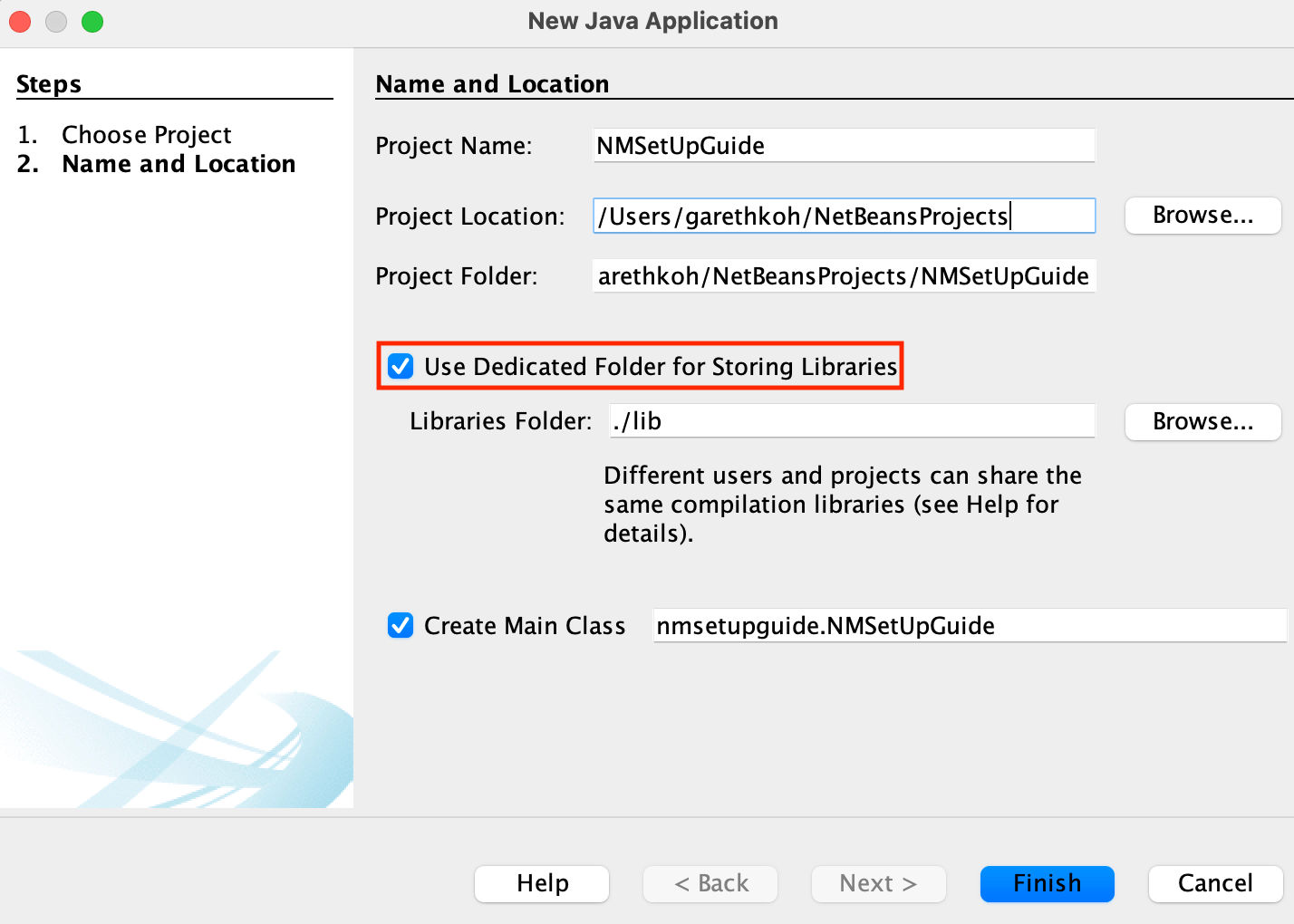We need to tell this Java project that we will be calling the NM Dev library. To do so, right click on the ‘Libraries’ Folder in the project tab and click ‘Add JAR/Folder’Browse to where you saved nmdev.jar. Select nmdev.jar and then hit ‘Open’.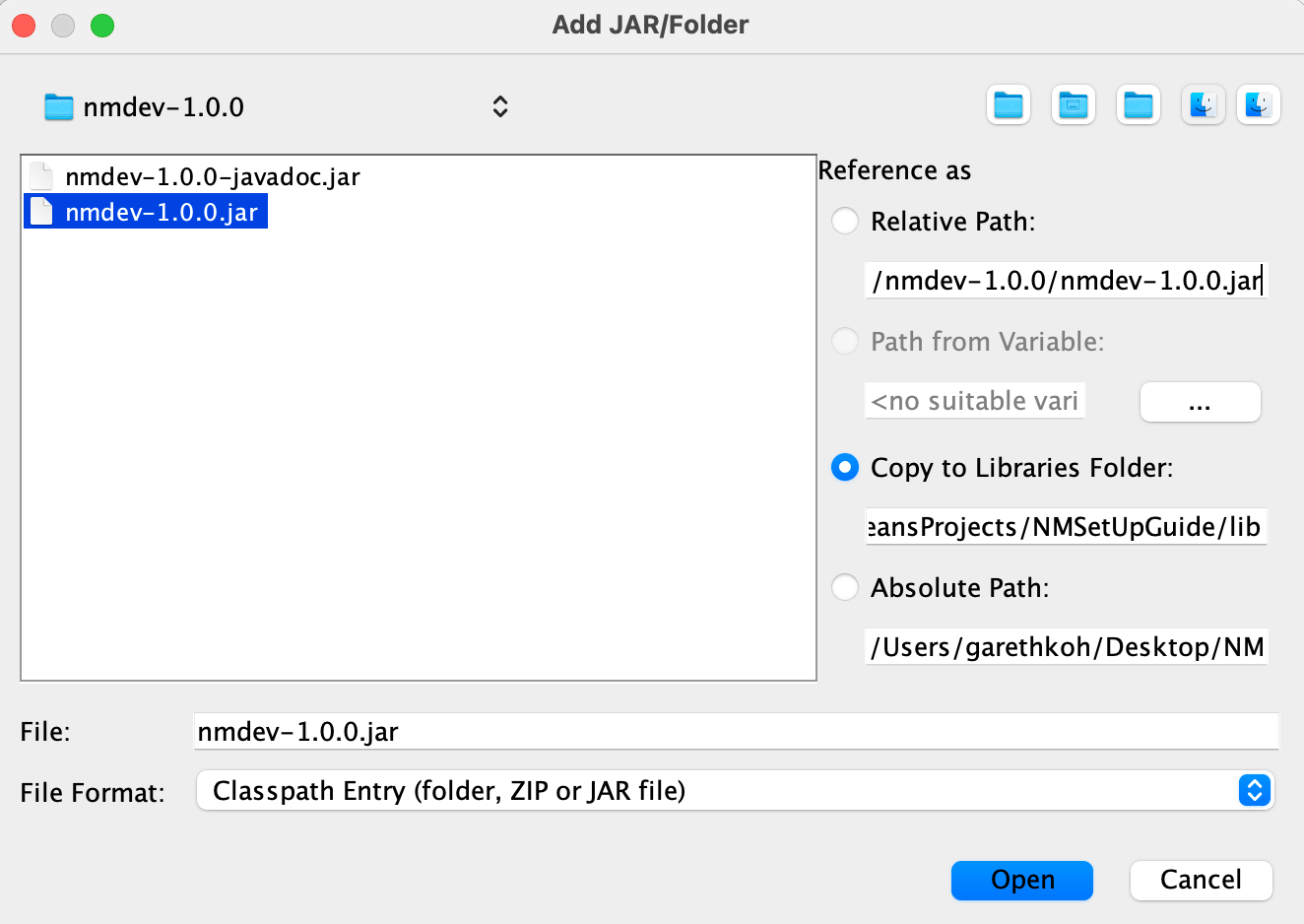To install the NM Dev javadoc in NetBeans, we need to associate the nmdev.jar with its javadoc. Right click the nmdev.jar file you just added and select ‘Edit’.In the popup window, under the ‘javadoc’ section, click ‘Browse’ and select the nmdev-javadoc.jar file. Then click ‘Open’.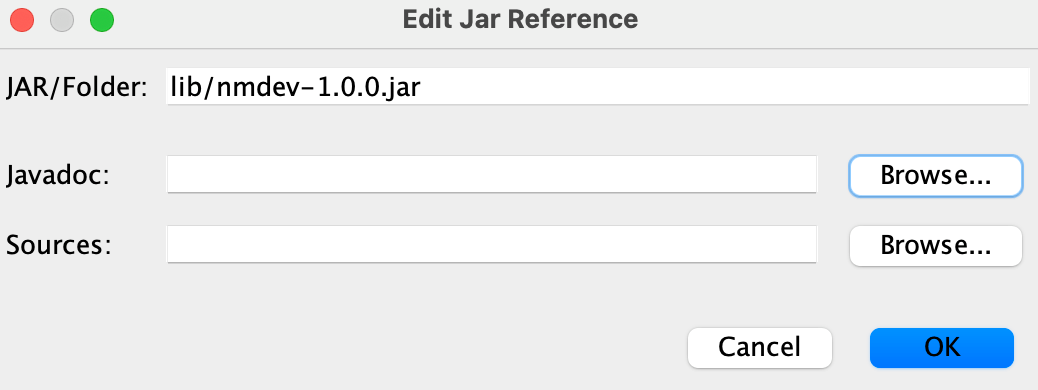Now, you have created an empty NM Dev project. Copy and paste these lines under “// TODO code application logic here“.

System.out.println(“Hello NM Dev”);

Matrix A1 = new DenseMatrix(new double[][]{//create a matrix

{1, 2, 1},

{4, 5, 2},

{7, 8, 1}

});

System.out.println(A1);

Matrix B = new Inverse(A1);//compute the inverse of A1

Matrix I = A1.multiply(B);//this should be the identity matrix

System.out.println(String.format(“%s * %s = %s (the identity matrix)”,

A1,

B,

I));

[/box]Hit Command+Shift+I (or Ctrl+Shift+I on Windows/Linux) to fix the necessary imports. Make sure these statements appear at the top of your program. Else you can input them manually.

import dev.nm.algebra.linear.matrix.doubles.Matrix;
import dev.nm.algebra.linear.matrix.doubles.matrixtype.dense.DenseMatrix;
import dev.nm.algebra.linear.matrix.doubles.operation.Inverse;

[/box]

Hit Alt+Shift+F to beautify your code. Your code should look something like this.Before you can run your code you will have to place your license file by doing

[/box]Right click on the project name, e.g., HelloNMDev, and click ‘Run’ (or simply press F6 if you make this project your main project). Voila! You should see this in your output window (Ctrl+4).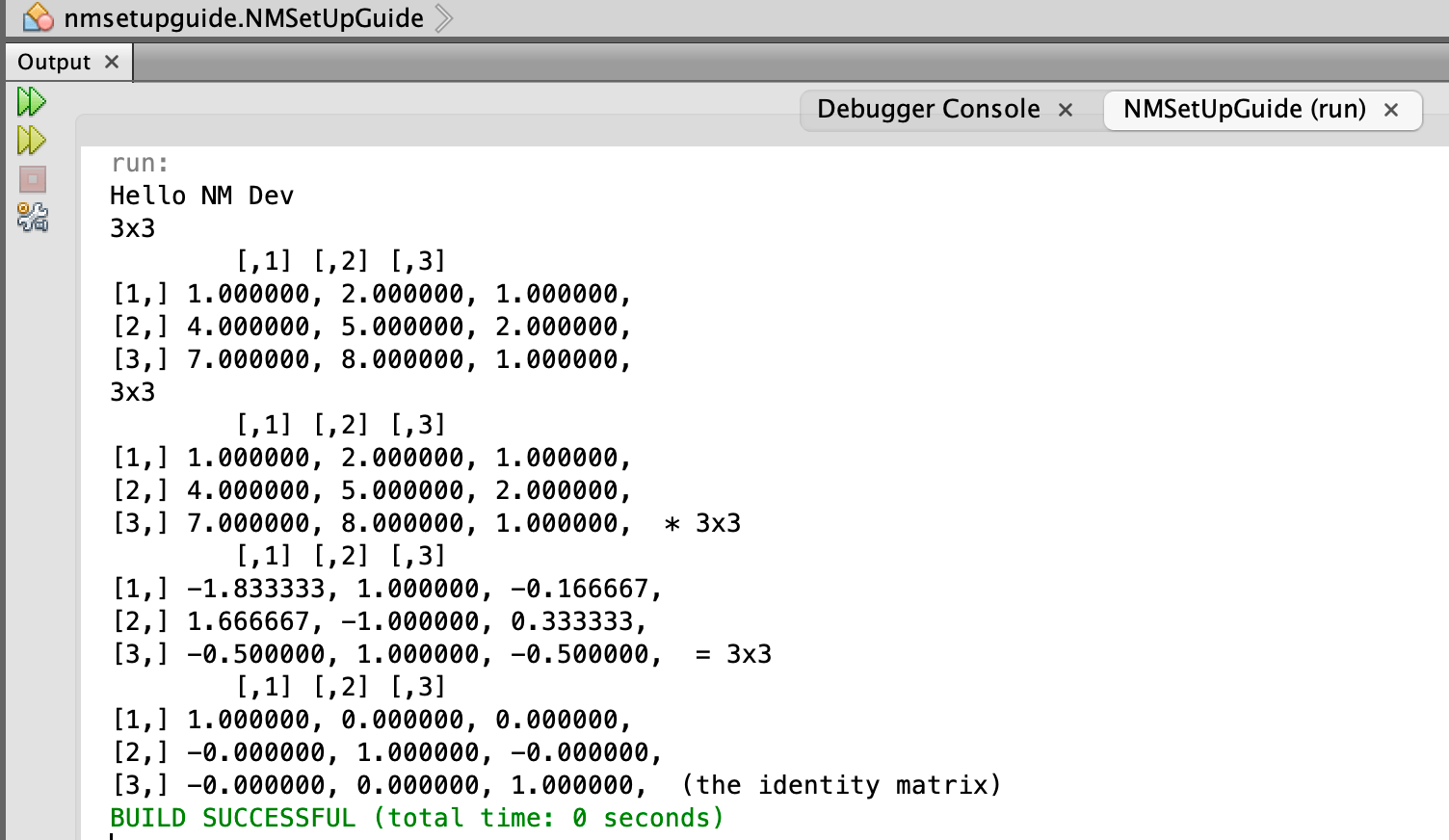Congratulations!

NM Dev’s javadoc is the complete reference to the NM Dev library. You can read it in a browser, e.g., Internet Explorer. You can bring them up dynamically during programming. Each time you press Ctrl+SPACE after the ‘.’ of any object you create, you will see a list of available methods for this object, and the javadoc for the method selected.# Hello NM Dev

This tutorial walks you through a simple first example on using the NM Dev library. You will need to have a NM Dev project to follow the examples here. For details on how to create or download a NM Dev project, please read the Setup Guide.

We will create some matrices and vectors and do some simple linear algebra. Java is an object-oriented language. So, other than the primitive types, like int and double, all variables are objects, namely, instances of some object definitions called classes. For example, to have a matrix instance, a matrix variable, or simply a matrix, we create an instance of the Matrix class.

Java matrix
Matrix A1 = new DenseMatrix(
new double[][]{ // create a matrix
{1, 2, 3},
{4, 5, 6},
{7, 8, 9}
});

We can print out the Java matrix to check.

System.out.println(A1);

In NetBeans the short cut for System.out.println is to type “sout” (without the “”) and then hit the “Tab” key.

To fix the imports, hit “Ctrl+Shift+I”. There are multiple choices. Choose com.numericalmethod.suanshu.matrix.doubles.Matrix.Hit “F6” to run.

Vola! You will see this output.

[,1] [,2] [,3]
[1,] 1.000000, 2.000000, 3.000000,
[2,] 4.000000, 5.000000, 6.000000,
[3,] 7.000000, 8.000000, 9.000000,

Congratulations! You have just successfully created your first matrix using the NM Dev library. We are now ready to do more.

Let’s check whether this matrix has a full rank. We do

int rank1 = MatrixMeasure.rank(A1); // compute A1's rank
System.out.println(rank1); // rank1 is 2 implies a deficient matrix

The MatrixMeasure.rank method computes the numerical rank. For more information, see Compute the numerical rank of a matrix.

Hit “F6” to run.

We see that the rank of A1 is 2 so A1 is a rank deficient matrix. We can make it a full rank matrix by changing one entry.

A1.set(3, 3, 10); // change A1[3, 3] to 10

Note that NM Dev counts from 1!

Let’s try again.

rank1 = MatrixMeasure.rank(A1); // compute modified A1's rank
System.out.println(String.format("rank for the modified A1 = %d", rank1));

OK. A1 is now a full rank matrix. We can now find its inverse.

Matrix A2 = new Inverse(A1); // compute A1 inverse
System.out.println(A2);

Hit “Ctrl+Shift+I” to fix the imports. Hit “F6” to run. Here is the output.

3x3

[,1] [,2] [,3]
[1,]  -0.666667, -1.333333, 1.000000,
[2,]  -0.666667, 3.666667, -2.000000,
[3,]  1.000000, -2.000000, 1.000000,

It looks good. To make sure, we multiply them together.

Matrix A3 = A1.multiply(A2); // A3 = A1 %*% A2
/** In numerical computing, we are usually satisfied to compare equality up to a precision.*/
final double precision = 1e-14; // A3 and the 3x3 identity matrix are equal up to 14 decimal points
if (AreMatrices.equal(A3, new DenseMatrix(3, 3).ONE(), precision)) { // compare A3 to the 3x3 identity matrix
System.out.println("A2 is indeed A1's inverse");

Hit “Ctrl+Shift+I” to fix the imports. Hit “F6” to run.

OK. It works.

Let’s try a vector. We do

Vector y = new DenseVector(new double[]{ // create a vector
20, 10, 4
});

Hit “Ctrl+Shift+I” to fix the imports. There are multiple choices. Choose “com.numericalmethod.suanshu.vector.doubles.Vector”.We solve a linear equation of this form.

Ax = y

We need to set up a linear equation solver.

LinearSystemSolver solver = new LinearSystemSolver(A1); // set up the linear equation solver
Vector x = solver.solve(y); // solve A1 %*% x = y
System.out.println(x);

Hit “Ctrl+Shift+I” to fix the imports. There are multiple choices. Choose com.numericalmethod.suanshu.matrix.doubles.linearsystem.LinearSystemSolver.

x is a vector

[-22.666667, 15.333333, 4.000000]

# The NM Dev Tutorials

The NM Dev tutorials are practical guides for those who would like to create mathematics applications using the NM Dev library. They include lessons with many complete and working examples. Groups of related lessons are organized into “trails”.

For the most up-to-date information on using the NM Dev library, please consult the Javadoc. Please check out the NM Forum, the place to discuss the NM Dev library, mathematics and programming. You are also encouraged to post your questions there to get help beyond documents.

Before you start these lessons, please make sure you have set up the development environment properly. You can find the details in the Setup Guide.

## Time Series Analysis

• various moving average filters
• univariate/multivariate time series analysis
• ARIMA modeling, simulation, fitting, prediction
• GARCH modeling, simulation, fitting, prediction
• Auto-Covariance computation

## Linear Regression

• OLS regression
• logistic regression
• GLM regression
• family: Binomial, Gamma, Gaussian, Inverse Gaussian, Poisson
• quasi-family: Binomial, Gamma, Gaussian, Inverse Gaussian, Poisson
• GLM model selection

## Hypothesis Testing

• Kolmogorov-Smirnov
• D’Agostino
• Jarque-Bera
• Lilliefors
• Shapiro-Wilk
• One-way ANOVA
• T
• Kruskal-Wallis
• Siegel-Tukey
• Van der Waerden
• Wilcoxon rank sum
• Wilcoxon signed rank
• Breusch-Pagan
• Glejser
• Harvey-Godfrey
• White
• Bartlett
• Brown-Forsythe
• F
• Levene

## STATS

This is a collection of advanced statistics algorithms.

• cointegration
• covariance selection
• factor analysis
• hidden Markov model (Rabiner)
• hidden Markov model (mixture models: Beta, Binomial, Exponential, Gamma, Log-Normal, Normal, Poisson)
• hypothesis testing
• Kalman filter
• LASSO (Least Absolute Shrinkage and Selection Operator)
• linear regression
• principal component analysis
• stochastic process simulation
• time series analysis

## OPTIM

This is a collection of the advanced optimization algorithms.

• linear programming (LP)
• quadratic programming (QP)
• second order conic programming (SOCP)
• semi-definite programming (SDP)
• sequential quadratic programming (SQP)
• mixed linear integer programming (MIP)
• genetic algorithms (GA)
• differential evolution optimization (DE)

## OPDE

This is a collection of numerical algorithms to solve ordinary and partial differential equation problems.

• Ordinary Differential Equation (ODE) solvers for initial value problem (IVP):
• Euler’s method
• Runge Kutta
• 1st order Runge Kutta
• 2nd order Runge Kutta
• 3rd order Runge Kutta
• 4th order Runge Kutta
• 5th order Runge Kutta
• 6th order Runge Kutta
• 7th order Runge Kutta
• 8th order Runge Kutta
• 10th order Runge Kutta
• Runge-Kutta-Fehlberg (RKF45) (adaptive step-size control)
• Adams-Bashforth-Moulton predictor-corrector multi-step method
• 1st order
• 2nd order
• 3rd order
• 4th order
• 5th order
• solvers based on Richardson extrapolation
• Burlisch-Stoer extrapolation
• semi-implicit extrapolation (suitable for stiff systems)
• first order system of ODEs
• conversion from high order ODE to first order ODE system
• Partial Differential Equation (PDE) solvers
• finite difference methods:
• elliptic problem:
• iterative central difference method (for Poisson’s equation)
• 1D hyperbolic problem:
• explicit central difference method (for 1D wave equation)
• 2D hyperbolic problem:
• explicit central difference method (for 2D wave equation)
• 1D parabolic problem:
• Crank-Nicolson method (for 1D heat or diffusion equation)
• 2D parabolic problem:
• alternating direction implicit (ADI) method (for 2D heat or diffusion equation)

## Packages

NM Dev is a large collection of numerical algorithms covering many topics in mathematics. The following is a summary of our packages. For a complete listing, please refer to the Javadoc.

• BASIC: basic numerical analysis algorithms
• OPTIM: advanced optimization algorithms
• STATS: advanced statistics algorithms
• O/PDE: ordinary and partial differential equations
• DSP: digital signal processing
• EVT: extreme value theory
• many more……

All our code is well tested. We have thousands of test cases that run daily.

Disclaimer: For each subject/topic heading above, there may or may not be features you expected. You will have to download the trial version to experiment with the library to decide whether it is suitable for your purpose, or whether the features you are looking for are included in the library or a particular version of the library. For example, we have listed “OLS regression” in the feature list, but not all possible mathematics, subjects, or topics related to OLS regression are found in the NM Dev library.